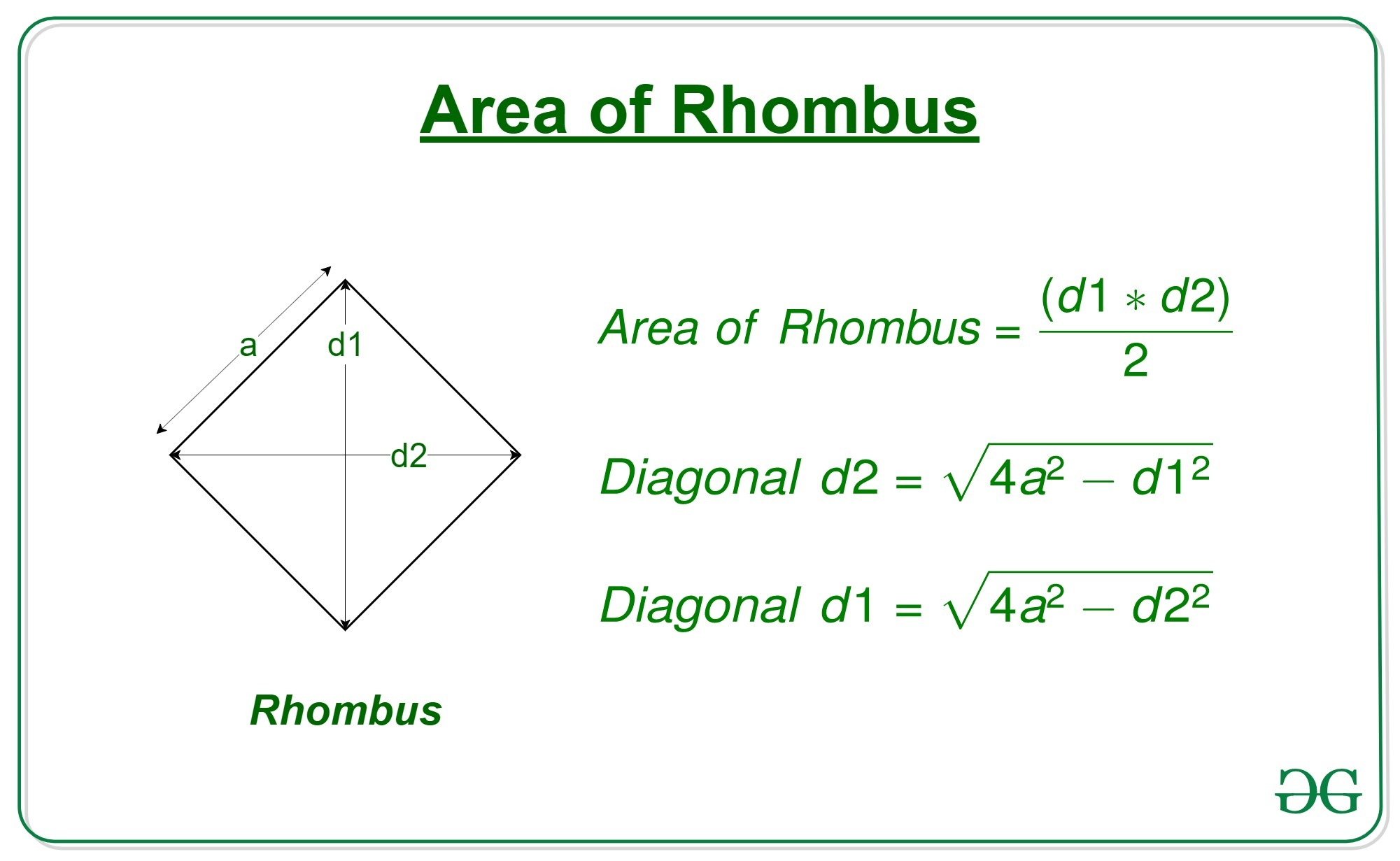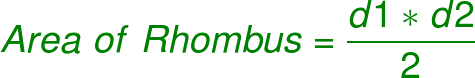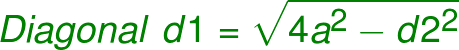# Program to calculate area of a rhombus whose one side and diagonal are given

Given the length of diagonal ‘d1’ of a rhombus and a side ‘a’, the task is to find the area of that rhombus.

A rhombus is a polygon having 4 equal sides in which both the opposite sides are parallel, and opposite angles are equal.Examples:

```Input: d = 15, a = 10
Output: 99.21567416492215

Input: d = 20, a = 18
Output: 299.3325909419153```

Approach:

• Get the diagonal ‘d1’ and side ‘a’ of the rhombus
• We know that,•
• But since we don’t know the other diagonal d2, we cannot use this formula yet
• So we first find the second diagonal d2 with the help of d1 and a•
• Now we can use the area formula to compute the area of the Rhombus

## C++

 `// C++ program to calculate the area of a rhombus` `// whose one side and one diagonal is given` `#include` `using` `namespace` `std;`   `// function to calculate the area of the rhombus` `double` `area(``double` `d1, ``double` `a)` `{` `    `  `    ``// Second diagonal` `    ``double` `d2 = ``sqrt``(4 * (a * a) - d1 * d1);`   `    ``// area of rhombus` `    ``double` `area = 0.5 * d1 * d2;`   `    ``// return the area` `    ``return` `area;` `}`   `// Driver code` `int` `main()` `{` `    ``double` `d = 7.07;` `    ``double` `a = 5;` `    ``printf``(``"%0.8f"``, area(d, a));` `}`   `// This code is contributed by Mohit Kumar`

## Java

 `// Java program to calculate the area of a rhombus` `// whose one side and one diagonal is given` `class` `GFG` `{`   `    ``// function to calculate the area of the rhombus` `    ``static` `double` `area(``double` `d1, ``double` `a)` `    ``{` `        `  `        ``// Second diagonal` `        ``double` `d2 = Math.sqrt(``4` `* (a * a) - d1 * d1);` `    `  `        ``// area of rhombus` `        ``double` `area = ``0.5` `* d1 * d2;` `    `  `        ``// return the area` `        ``return` `area;` `    ``}` `    `  `    ``// Driver code` `    ``public` `static` `void` `main (String[] args) ` `    ``{` `        ``double` `d = ``7.07``;` `        ``double` `a = ``5``;` `        ``System.out.println(area(d, a));` `    ``}` `}`   `// This code is contributed by AnkitRai01`

## Python3

 `# Python program to calculate` `# the area of a rhombus` `# whose one side and ` `# one diagonal is given`   `# function to calculate ` `# the area of the rhombus` `def` `area(d1, a):` `    `  `    ``# Second diagonal` `    ``d2 ``=` `(``4``*``(a``*``*``2``) ``-` `d1``*``*``2``)``*``*``0.5` `    `  `    ``# area of rhombus` `    ``area ``=` `0.5` `*` `d1 ``*` `d2` `    `  `    ``# return the area` `    ``return``(area)`   `# driver code` `d ``=` `7.07` `a ``=` `5` `print``(area(d, a))`

## C#

 `// C# program to calculate the area of a rhombus` `// whose one side and one diagonal is given` `using` `System;`   `class` `GFG` `{`   `    ``// function to calculate the area of the rhombus` `    ``static` `double` `area(``double` `d1, ``double` `a)` `    ``{` `        `  `        ``// Second diagonal` `        ``double` `d2 = Math.Sqrt(4 * (a * a) - d1 * d1);` `    `  `        ``// area of rhombus` `        ``double` `area = 0.5 * d1 * d2;` `    `  `        ``// return the area` `        ``return` `area;` `    ``}` `    `  `    ``// Driver code` `    ``public` `static` `void` `Main (String []args) ` `    ``{` `        ``double` `d = 7.07;` `        ``double` `a = 5;` `        ``Console.WriteLine(area(d, a));` `    ``}` `}`   `// This code is contributed by Arnab Kundu`

## Javascript

 ``

Output:

`24.999998859949972`

Time Complexity: O(log(n)) as inbuilt sqrt function is used

Auxiliary Space: O(1)

Feeling lost in the world of random DSA topics, wasting time without progress? It's time for a change! Join our DSA course, where we'll guide you on an exciting journey to master DSA efficiently and on schedule.
Ready to dive in? Explore our Free Demo Content and join our DSA course, trusted by over 100,000 geeks!